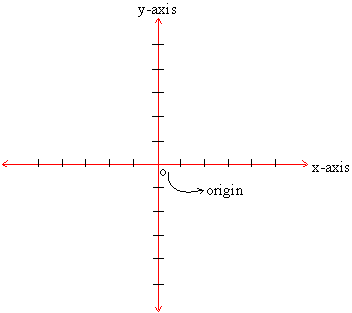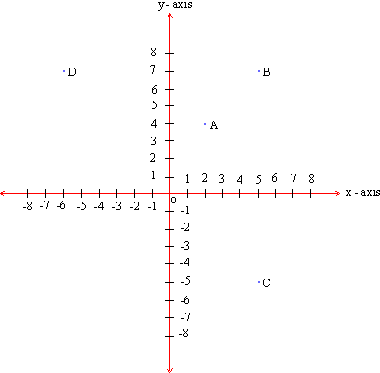Home MonkeyNotes Printable Notes Digital Library Study Guides Message Boards Study Smart Parents Tips College Planning Test Prep Fun Zone Help / FAQ How to Cite New Title Request

 9.2 Co - ordinates and Axes In order to indicate the location of the point P, if we decide to write the horizontal units first and the vertical units next, then the position of the point P can be expressed as P ( 4, 34 ). This pair of numbers is called co-ordinates of the point P ; and they entirely describe the position of point P in the plane LMNO with respect to the reference point 0. Hence, the location of each point in a plane can be expressed with a pair of numbers called the co-ordinates of that point. The horizontal line is called the x-axis and the vertical line is called the y-axis. The reference point is called the origin. The x-axis and y-axis are co-planar. These points as well as the points in their plane are called a co-ordinate plane ( figure 9.2)Figure 9.2 X - Co-ordinate : While determining the position of a point in the co-ordinate plane, the value of the horizontal unit is called the x-co-ordinate of that point. This value is positive if measured to the right of the origin and negative if measured to the left of the origin. Y- Co-ordinate : While determining the position of a point in the co-ordinate plane, the value of the vertical unit is called the y-co-ordinate of that point. This value is positive if measured upwards from the origin and is negative if measured downwards from the origin. Ordered Pair : In order to name a point in the co-ordinate plane, the x and y co-ordinates of that point have to be written (first x-co-ordinate then y-co-ordinate) in brackets / parentheses separated by a coma. This pair of numbers is called the ordered pair. For e.g. if the x and y-co-ordinates of a point A are 3 and 5 respectively then the ordered pair to describe A is written as A (3, 5). The ordered pair for the origin is (0, 0). Example 1 Identify the co-ordinates of the points A, B, C & D in the following figure and write them in their ordered pairs.Solution : A ( 2, 4 ) B ( 5, 7 ) C ( 5, -5 ) D ( -6, 7 ) Index 9.1 Points And Co-ordinates 9. 2 Co-ordinates and Axes 9. 3 Quadrants 9. 4 Distances And Distances Formula 9. 5 Mid Point Formula 9. 6 Slope Of A Line 9. 7 Equation Of A Line Chapter 1
 Search: All Products Books Popular Music Classical Music Video DVD Toys & Games Electronics Software Tools & Hardware Outdoor Living Kitchen & Housewares Camera & Photo Cell Phones Keywords: